# Negative dynamic mass density and locally resonant sonic materials

### Negative dynamic mass density for composites

Mass density of a composite D is generally regarded as the volume average of its components’densities and hence must be positive. One application of the mass density is in predicting the wave velocity: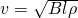, where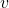is the longitudinal wave velocity, B the bulk modulus, and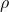the dynamic mass density. The question is: Does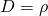necessarily for all composites? We have shown through rigorous derivation with experimental support that the correct answer to the above question is “No.” Moreover,can be negative when there is a resonance in the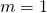angular channel of wave scattering. What that implies is that there can indeed be a one-to-one correspondence between the electromagnetic metamaterials, in which both the electric and magnetic permeabilities are negative, and the acoustic metamaterials, in which both the dynamic mass density and bulk modulus are negative. In fact, the negative dynamic mass density was realized through the locally resonant sonic materials, shown below.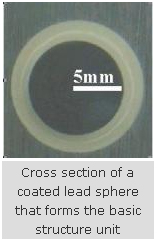Key publications:

1. Effective Dynamic Mass Density of Composites“, J. Mei, Z. Liu, W. Wen and Ping Sheng, Phys. Rev. B 76, 134205 (2007).
2. Dynamic Mass Density and Acoustic Metamaterials“, J. Mei, Z. Liu, W. Wen and Ping Sheng, Physica B 394, 256-261 (2007).
3. Effective Mass Density of Fluid-Solid Composites“, J. Mei, Z. Liu, W. Wen and Ping Sheng, Phys. Rev. Lett. 97, 044501 (2006).
4. Analytic Model of Phononic Crystals with Local Resonances“, Z. Liu, C. T. Chan and Ping Sheng, Phys. Rev. B71, 014103 (2005).

### Locally resonant sonic materials

The locally resonant sonic material is designed to reject completely sound waves at a pre- specified range of frequencies. The perfect reflection is achieved by creating elastic wave band gaps induced by local resonances. Conventional elastic wave band gap material operates under the principle of Bragg reflection, which dictates that the thickness of the slab must be a few times of the sound wave to shield. This makes Bragg-reflection based band gap material too bulky for low frequency applications since low frequency sound has long wavelength. Our material has sonic band gaps by virtue of the vibrational resonance of certain structural unit(s). There are several advantages of using resonance to create a band gap. First, periodicity is not required (in contrast to Bragg reflection). Second, the resonance frequency has nothing to do with the lattice constant, and hence the slab can in principle be much thinner than the wavelength of the sound it is supposed to shield.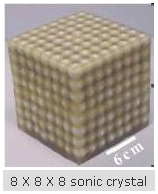This unit has a low frequency resonance, at 400 Hertz, plus a higher frequency resonance. When assembled together in the form of a simple cubic sonic crystal, the measured sonic transmission exhibits a sonic gap at 400 Hertz. This is remarkable, since the wavelength of 400 Hertz sound wave in the matrix material is about 300 times the lattice constant. Thus away from the resonance, the material can be homogenized, and the elastic waves do not “see” the structural units. However, close to the resonances, the large dispersion of the response function (similar to a very large index of refraction for light) means that the effective wavelength is much smaller and becomes comparable to the lattice constant, hence the strong scattering. This peculiar material can break the so-called “mass law” of sound transmission. It should be noted that an attenuation gap can be due either to negative elastic constant or to negative dynamic mass density. In the publication below, the effect was wrongly attributed to negative elastic constant, but this has been corrected in the subsequent publications (see above).

Key publication:

1. Locally Resonant Sonic Materials“, Zhengyou Liu, Xixiang Zhang, Yiwei Mao, Y. Y. Zhu, Zhiyu Yang, C. T. Chan, and Ping Sheng, Science 289, 1734-1736.